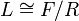Exterior square of a Lie ring

Suppose$L$ is a Lie ring. The exterior square of$L$, denoted$L \wedge L$ or$\bigwedge^2 L$, can be defined in the following equivalent ways:
1. It is the exterior product of$L$ with itself, viewing it as two copies of itself in itself (in general, the exterior product is defined for two possibly equal ideals inside a Lie ring).
2. It is the derived subring of any Schur covering Lie ring of$G$. Note that the Schur covering Lie rings need not be isomorphic Lie rings, but they are isoclinic Lie rings, so the definition is independent of the choice of Schur covering Lie ring.
3. If$L \cong F/R$ where$F$ is a free Lie ring and$R$ is an ideal in$F$, it is, up to isomorphism, the same as$[F,F]/[F,R]$.